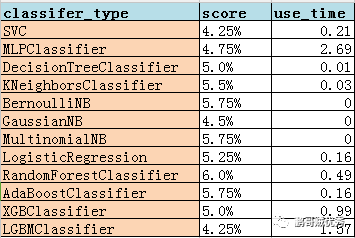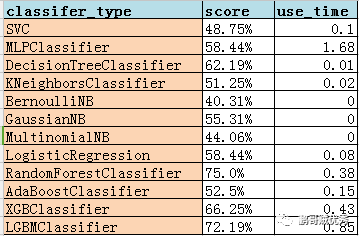# 【华为云技术分享】【Python成长之路】机器学习：10+分类算法汇总学习(示例代码)

## 示例代码

```  1 from sklearn import svm
2 from sklearn.neural_network import MLPClassifier
3 from sklearn.tree import DecisionTreeClassifier
4 from sklearn.neighbors import KNeighborsClassifier
5 from sklearn.naive_bayes import BernoulliNB
6 from sklearn.naive_bayes import GaussianNB
7 from sklearn.naive_bayes import MultinomialNB
8 from sklearn.linear_model import LogisticRegression
9 from sklearn.ensemble import RandomForestClassifier
10 from sklearn.ensemble import AdaBoostClassifier
11 import xgboost
12 import lightgbm
13 import time
14 from sklearn import metrics
15 from sklearn.model_selection import train_test_split
16 import random
17 import numpy
18 from openpyxl import Workbook,load_workbook
19
20
21 def train_test_algorithm(model, x_train, y_train, x_test, y_test):
22     print(‘begin to train……‘)
23     start_time = time.time()
24     model.fit(x_train, y_train)
25     print(‘begin to predict……‘)
26     y_pred_model = model.predict(x_test)
27     classifier = str(model).split(‘(‘)
28     print(‘begin to get the result of Classifier_Type:{}...‘.format(classifier))
29     score = metrics.accuracy_score(y_test, y_pred_model)
30     print(‘The accuracy score of {0} is: {1}%‘.format(classifier, round(score * 100, 2)))
31     end_time = time.time()
32     use_time = end_time - start_time
33     print(‘The time of using {0} is :{1}‘.format(classifier, round(use_time, 2)))
34     print(‘{} test end!

‘.format(classifier))
35     return score, round(use_time, 2), classifier
36
37
38 def get_redball():
39     redlist = []
40     while len(redlist) < 7:
41         temp = random.randint(1, 34)
42         if temp not in redlist:
43             redlist.append(temp)
44     return redlist
45
46
47 def get_blueball():
48     return random.randint(1, 17)
49
50
51 def get_data():
52     x_temp, y_temp = [], []
53     for i in range(2000):
54         x_temp.append(get_redball())
55         y_temp.append(get_blueball())
56     x = numpy.array(x_temp)
57     y = numpy.array(y_temp)
58     return x, y
59
60 def get_data2():
61     import csv
62     data = csv.reader(open(‘winequality-red.csv‘,‘r‘))
63     labels,xlist=[],[]
64     firstline = True
65     for i in data:
66         if firstline:
67             firstline = False
68         else:
69             r = i.split(‘;‘)
70             labels.append(float(r[-1]))
71             r.pop()
72             floatrow = [float(num) for num in r]
73             xlist.append(floatrow)
74     # 转化为numpy格式
75     x = numpy.array(xlist)
76     y = numpy.array(labels)
77     return x,y
78
79
80 if __name__ == ‘__main__‘:
81     # 定义各类分类算法
82     svc = svm.SVC()  # 支持向量机，SVM
83     mlp = MLPClassifier()  # 多层感知机，MLP
84     dtc = DecisionTreeClassifier()  # 决策树,DT
85     knc = KNeighborsClassifier()  # K最近邻,KNN
86     bnb = BernoulliNB()  # 伯努利贝叶斯，BNB
87     gnb = GaussianNB()  # 高斯贝叶斯,GNB
88     mnb = MultinomialNB()  # 朴素贝叶斯，MNB
89     lgr = LogisticRegression()  # 逻辑回归，LGR
90     rfc = RandomForestClassifier()  # 随机森林，RFC
91     abc = AdaBoostClassifier()  # AdaBoost
92     xgb = xgboost.XGBClassifier()  # xgboost
93     gbm = lightgbm.LGBMClassifier()  # lightgbm
94
95     # 训练/测试数据生成
96     # 真实红酒销售数据
97     x, y = get_data2()
98     # 随机生成的双色球红蓝球数据
99     # x,y = get_data()
100     x_train, x_test, y_train, y_test = train_test_split(x, y, test_size=0.20, random_state=531)
101     classify_type_list = [svc, mlp, dtc, knc, bnb, gnb, mnb, lgr, rfc, abc, xgb, gbm]
102     test_result = {}
103     tablename = ‘wine_result‘
104     wb = load_workbook(‘result.xlsx‘)
105     try:
106         ws = wb[tablename]
107     except:
108         ws = wb.create_sheet(tablename)
109     ws.append([‘classifer_type‘,‘score‘,‘use_time‘])
110     for i, type in enumerate(classify_type_list):
111         score, use_time, classifier = train_test_algorithm(type, x_train, y_train, x_test, y_test)
112         ws.append([classifier,str(round(100*score,2))+‘%‘,use_time])
113     wb.save(‘result.xlsx‘)```

（1）双色球数据测试结果：（2）红酒质量数据测试结果：## 知识串讲

（1）分类算法代码实现流程：

1、准备数据样本，通过train_test_split函数可自动按比例生成训练和测试的数据数量，如样例代码为20%的测试数据，80%的训练数据；

2、实例化具体某个算法，如mlp = MLPClassifier();

3、通过fit函数对训练数据(x_train/y_train)进行预处理；

4、基于测试x_test数据进行具体算法的预测（predict函数实现），产生y_pred_data；

5、将预测数据y_pred_data与真实数据y_test进行对比，可以通过平方差等各类评估方法来判定，分类算法的准确率。

（2）示例代码有一部分代码是涉及excle表（csv/xlsx格式等）的处理，常用的python库有csv、openpyxl、xlrd等。因此我准备下次对excle表处理再进行汇总整理。这次就不具体记录了。

## 分类算法对比总结

K最近邻（KNN） 通过训练数据与预测数据之间的最邻近K个实例来判断，如果多数实例属于某个类别则预测数据属于那一类 优点：可以处理多分类问题，简单易懂 缺点：每次分类需要重新计算训练数据和测试数据，效率低；对训练数据的容错性较差，对多维数据无法处理

AdaBoost 将多个弱分类器通过加权结合，使其成为一个强分类器 优点：泛化误差低，不易过拟合，易与其他分类算法结合使用 缺点：对异常点非常敏感，并在分错后会逐级影响后面的弱分类器

xgboost 在GBDT（梯度提升决策树）基础发展而来，利用cpu的多线程引入正则化项 优点：不易过拟合，计算有提升，支持线性分类，能通过自动学习解决样本缺失问题 缺点：数据规格大时贪心算法比较耗时，对cache优化不友好

lightgbm 梯度boosting框架的升级版，使用基于学习算法的决策树，能通过减少数据并行过程中的通讯开销提升效率，目前貌似是最热闹的boosting算法 优点：训练效率快，支持并行化学习，能处理大规格数据。速度比xgboost更快

## 结束

```1 base_score=0.5, booster=‘gbtree‘, colsample_bylevel=1,
2               colsample_bynode=1, colsample_bytree=1, gamma=0,
3               learning_rate=0.1, max_delta_step=0, max_depth=3,
4               min_child_weight=1, missing=None, n_estimators=100, n_jobs=1,
5               nthread=None, objective=‘binary:logistic‘, random_state=0,
6               reg_alpha=0, reg_lambda=1, scale_pos_weight=1, seed=None,
7               silent=None, subsample=1, verbosity=1```

【华为云技术分享】Python 面向对象三大特征之多态(示例代码)

【华为云技术分享】华为开发者大会HDC.Cloud带你探索强化学习三大挑战及落地实践(示例代码)

【华为云技术分享】小白篇，认识Python最最最常用语重要的库Requests

【华为云技术分享】《跟唐老师学习云网络》 - Veth网线(示例代码)